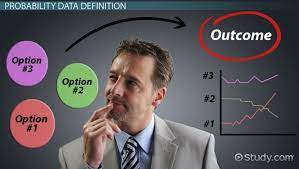(How Dr. Bongo Adi Applied Bayes’ Theorem to treat General Oleksiy Petro)

## Bayes’ Theorem

The theorem was developed by the popular English mathematicians and philosopher, Thomas Bayes. It was conceived to guide the new way of thinking about data and probability. It provides means of revising the prior probability based on any condition using either a table, a formula, or a Probability Tree. The simplest of the three is the probability table because it helps to simplify the interrelationship between the prior probability, the conditional probability, and the joint probability.

The Basis

In part one, we highlighted that multiplication law is used to determine the joint probability of two events P(A∩B). The additional law determines the conditional probability and is denoted as the probability of A, given the occurrence of B. The formular for conditional probability remains P(A|B) = P (A ∩ B)/P(B). An event is mutually exclusive when the joint probability is zero P(A B)=0. The marginal probability is the average of the conditional probability over the distribution of all values of the given event. We would be looking at the application of Bayes’ theorem in providing a basis for making critical business decisions..

## Application of Bayes’ Theorem: Dr. Bongo Adi and Ukrainian General

Assuming Dr. Bongo Adi diagnosed an Ukrainian General Oleksiy Petro who flew to Nigeria for treatment after the deadly invasion of the country by the Russian military. The patient showed symptoms of having either trench fever or typhoid fever, denoted as T1 and T2 respectively. The probability of the patient having Trench fever (T1) was quantified to be 60% and 40% for typhoid fever (T2). The medical researcher, Dr. Francis Okoye assigned 15%, 10%, and 15% probability associated with the three symptoms (S1, S2, and S3) to trench fever. Also, he assigned 80%, 15%, and 3% probability that each of the three symptoms is related to typhoid fever (T2). Before Dr. Bongo could make any prescription on the treatment of the General ailment, he requested further medical diagnosis:

1. To determine the posterior probability of symptom S1
2. With the symptom S1 established in 1 above, supposing he also shows signs of symptom S2. What are the revived probabilities of trench fever (T1) and typhoid fever (T2) in the General?

## The revised or posterior probability of Symptom S1 by General Oleksiy

To determine the revised probability that General Oleksiy Petro shows symptom S1, the meticulous Dr. Francis took 5 steps to arrive at his conclusion:

1. He established the prior or initial probability as 0.6 for T1 and 0.4 for T2
2. He computed the conditional probability of the two events (S1/T1 or S1/T2). This he could have determined by dividing the joint probability by the prior probability of the event P(S1|T1)=P(S1∩T2)/P(T1). However, he adopted the data he provided earlier to Dr. Bongo as 0.15 for T1 and 0.8 for T2.
3. Dr. Francis calculated the joint probability of each of the two events (T1 and T2). He multiplied the conditional probability by the initial probability and prepared this on simple table. However, he remembered the formula as P(A∩B)=P(A|B)xP(B).
4. He added the result of the joint probability of the two events to determine the Marginal probability.
5. Most importantly, Dr. Francis provided the revised probability that summed up of 1. Doing this, he divided the joint probability of each event by the marginal probability.

Dr. Francis sent the simplified table below to Dr. Bongo with the understanding that the chance of the General suffering from Typhoid fever was high at 78%.

## The revised probability: General Oleksiy Symptom S1 and S2

As established, the revised probability of the General having Trench fever was 21.95% and Typhoid fever as 78.05%, given symptom1. Dr. Francis repeated the 5 steps above by considering the 21.95% and 78.05% determined initially as the prior probability. He computed this in consideration of the conditional probability of Symptoms2, given disease T1 and T2.

## Conclusion

Bayes’ theorem of revised probability is an important statistical tool that helps to improve the predicted probability of an event by incorporating new information. As in our example, Dr. Bongo Adi is now well equipped with more data to establish that General Oleksiy Petro is suffering from Typhoid Fever at 84% compare to the 15.79% for trench fever.

#EMBA27. #SEAL07## Next Generation Fixed Wireless Access (NGFWA) for Service Providers: A Viable Alternative to Traditional Fiber-Optic Networks

in## Transforming Nigeria’s Local Government System for Rural Economic Growth and Increased GDP

in